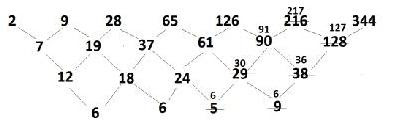# Number Series for IBPS CWE PO - VI Prelim Exam (Set-3)### Directions (1 - 3): Complete the following series.

1. 2, 4, 12, 4, 240, ?
a) 960
b) 1440
c) 1080
d) 1920
e) None of these
2. 2, 5, 9, 19, 37, ?
a) 76
b) 74
c) 75
d) 73
e) None of these

3. 4, -8, 16, -32, 64, ?
a) 128
b) -128
c) 192
d) -192
e) None of these

### Directions (4 - 5): Find the wrong term in the following given series.

4. 2, 9, 28, 65, 126, 216, 344
a) 2
b) 28
c) 65
d) 126
e) 216

5. 10, 26, 74, 218, 654, 1946, 5834
a) 26
b) 74
c) 218
d) 654
e) 1946

1. a) 2, 4, 12, 48, 240, ….
The pattern is: to arrive at a term, the previous term is being multiplied by (n+1) where ‘n’ keeps on increasing by 1 for every term.

2
4 = 2 × (2 + 0)
12 = 4 × (2 + 1)
48 = 12 × (2 + 2)
240 = 48 × (2 + 3)
⇒ Next term = 240 × (2 + 4) = 240 × 6 = 1440

2. c) 2, 5, 9, 19, 37, …..
The pattern is: every number is arrived at previous number multiplied by 2 and then alternate addition and subtraction by 1 i.e.
2
5=2×2+1
9=5×2-1
19=9×2+1
37=19×2-1
the next term 37×2+1 = 75

3. b) 4, -8, 16, -32, 64, ….
The pattern is: Every number is arrived at by multiplying previous alternate number with ‘4’ as shown below:
4*4 = 16
-8*4 = -32
16*4 = 64
-32*4 = -128
Hence, ‘-128’ is the correct answer.

4. e) 2, 9, 28, 65, 126, 216, 344.
The pattern in the series is that the series is triangular as shown below:In the triangular series, the difference between consecutive terms is written below the numbers and then, difference between consecutive differences is written below & this process carries on until all the difference become equal. In the figure above there was an error & we have corrected it.

5. d) 10, 26, 74, 218, 654, 1946, 5834
The pattern is: to arrive at next term, the previous is multiplied by 3 and subtracted by 4:
10
10 × 3 – 4 = 26
26 × 3 – 4 = 74
74 × 3 – 4 = 218
218 × 3 – 4 = 650 ≠ 654
650 × 3 – 4 = 1946
1946 × 3 – 4 = 5834
Here, ‘654’ was wrong.

Thanks.